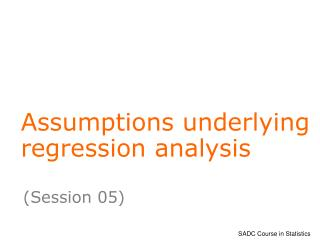DownloadDownload PresentationAssumptions underlying regression analysis

# Assumptions underlying regression analysis

Download Presentation## Assumptions underlying regression analysis

- - - - - - - - - - - - - - - - - - - - - - - - - - - E N D - - - - - - - - - - - - - - - - - - - - - - - - - - -
##### Presentation Transcript

1. Assumptions underlying regression analysis (Session 05)

2. Learning Objectives At the end of this session, you will be able to • describe assumptions underlying a regression analysis • conduct analyses that will allow a check on model assumptions • discuss the consequences of failure of assumptions • consider remedial action when assumption fail

3. Checking assumptions Describing the relationship carries no assumptions. However, inferences concerning the slope of the line, e.g. by use of t-tests or F-tests, are subject to certain assumptions. Checking assumptions is important to avoid pitfalls associated with making invalid conclusions.

4. Assumptions The simple linear regression model is: yi = 0 + 1 xi + i In addition to assuming a linear form for the model, the i are assumed to be • independent, with • zero mean and constant variance 2, • and be normally distributed. Note: Model predictions, often called fitted values, are

5. How to check assumptions? The usual approach is to conduct a residual analysis. Residuals are deviations of observed values from model fitted values. Paddy data relating yield to fertiliser Residual

6. Residual Plots Plotting residuals in various ways allows failure of assumptions to be detected. e.g. To check the normality assumption, • plot a histogram of the residuals (provided there are enough observations); • or do a normal probability plot of residuals. A straight line plot indicates that the normality assumption is reasonable.

7. Residual Plots - continued Most useful is a plot of residuals against fitted values( ) . It helps to detect failure of the variance homogeneity assumption. Also helps to identify potential outliers. e.g. If standardised residuals are used, i.e. residuals/standard error, then 95% of observations would be expected to lie between –2 and +2. A random scatter with no obvious pattern is good! Some examples follow….

8. Some Residual Plots x x x x x x x x x x x x x x 0 x x x x x x x x x x x A random scatter as above is good. It shows no obvious departures of the variance homogeneity assumption.

9. Some Residual Plots x x x x x x x x x x x x 0 x x x x x x x x x x x x x x Variance increases with increasing x. Could try a loge(y) transformation.

10. Some Residual Plots x x x x x x x x x x x x x x 0 x x x x x x x x x x x x Indication that the response is a binomial proportion. Use a logistic regression model.

11. Some Residual Plots x x x x x x x x x x x 0 x x x x x x x x x x x x x x Lack of linearity. Pattern indicates an incorrect model - probably due to a missing squared term.

12. Some Residual Plots x x x x x x x x x x x 0 x x x x x x x x x x x x x x Presence of an outlier. Investigate if there is a reason for this odd-point.

13. Consequences of assumption failure Studies on consequences of assumption failure have demonstrated that: • tests and confidence intervals for means are relatively robust to small departures from non-normality; • the effects of non-homogeneous variance can be large, but not so serious if sample sizes in different sub-groupings are equal; • dependence of observations can badly affect F-tests.

14. Dealing with assumption failure One approach is to find a transformation that will stabilize the variance. Some typical transformations are: • taking logs (useful when there is skewness); • square root transformation; • reciprocal transformation. Sometimes theoretical grounds will determine the transformation to use, e.g. when data are Poisson or Binomial. However, in such cases, exact methods of analysis will be preferable.

15. Dealing with non-independence The assumption of independence is quite critical. Some attention to this is needed at the data collection stage. If observations are collected in time or space, plotting residuals in time (or space) order may reveal that subsequent observations are correlated. Techniques similar to those used in time series analysis or analysis of repeated measurements data may be more appropriate.

16. An illustration – using paddy data Histogram of standardised residuals after fitting a linear regression of yield on fertiliser. This is a check on the normality assumption.

17. A normal probability plot… Another check on the normality assumption Do you think the points follow a straight line?

18. Std. residuals versus fitted values Checking assumption of variance homogeneity, and identification of outliers: Do you judge this to be a random scatter? Are there any outliers?

19. Conclusion: The residual plots showed no evidence of departures from the model assumptions. We may conclude that fertiliser does contribute significantly to explaining the variability in paddy yields. Note: Always conduct a residual analysis after fitting a regression model. The same concepts carry over to more complex models that may be fitted.